# 重写 500 Lines or Less 项目 - 3D 建模器

## 概述

### 背景

`3D Modeller` 基于 `Python` 语言和 `OpenGL` 实现了可在窗口中查看、编辑和浏览的三维模型场景，有点类似于一个简化版的 `3DS Max`。对于初次接触三维图形编程的读者来说，主要难点在于它涉及到比较多的空间几何知识和图形变换；在此基础上，需要了解的 `OpenGL` 接口也具有相当的规模和复杂度。我简单统计了一下，原文仅作者编写的代码已经超过 650 行，这还没有包括其所引用的两个第三方模块————这些模块被作者以源码形式包含在了项目中，而这两个模块加起来也有 500 行左右了。因此可以说，该项目在 500lines 系列中也算是规模和难度比较大的一个了。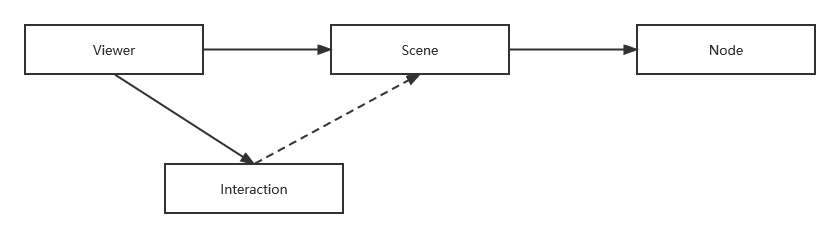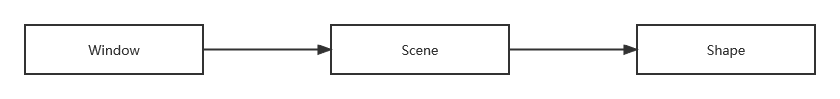### 目标

`3D Modeller` 是一个三维展示和设计程序。由于计算机屏幕本身是二维的（不考虑 `VR`），在目前的技术限制下，我们只能将三维空间的内容“投影”到二维平面上。二维平面的主要问题在于它无法直观地表示出“景深”的维度，所以在视觉上需要一些辅助内容来帮助我们进行空间定位，其中主要方法之一就是在三维设计应用中常见的空间网格线（如下图）。为了避免不必要的干扰，本例显示的网格只显示 X-Y 平面，同时标记出三个坐标轴方向。因此示例中把该线称为平面（`plane`）。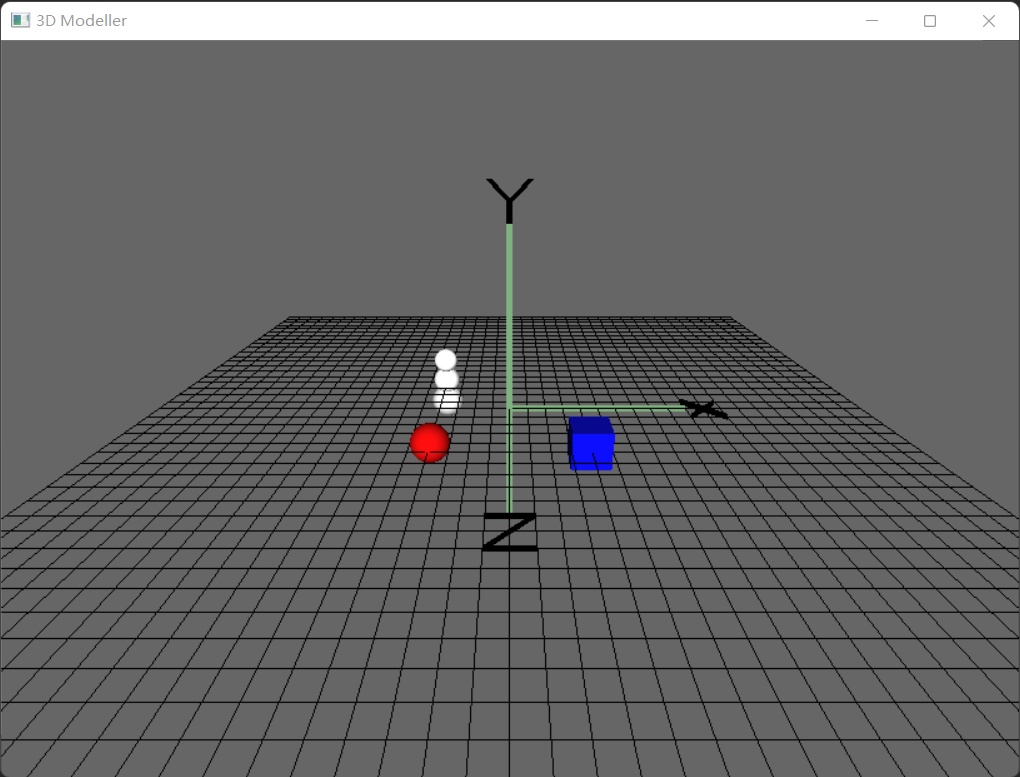• 球体（`Sphere`
• 方块（`Cube`
• 雪球（`Snowball`）。它实际上是一个复合形体，由三个小的圆球所构成，同时也是展示关于 `Composite` 界面设计模式的一个很好的实例。

• 鼠标左键点击形体进行选择（限于篇幅，程序只支持单选）。后续移动、缩放等操作都是对于选中的形体而言的
• 按住鼠标左键拖动，对选中的形状进行平移
• 按住鼠标中键拖动，对整个场景（也包括其中的形体）进行平移
• 按住鼠标右键拖动，则是以原点为中心对场景进行旋转
• 使用鼠标滚轮，对场景进行整体放大或缩小

• `s` 键，在鼠标位置放置一个新的球体
• `c` 键放置一个方块
• `b` 键放置一个雪球
• 按方向键上/下，将选中的形体放大或缩小
• 按方向键左/右，让选中形体的表面颜色在一系列预定义颜色中切换

### 源码

• `PyOpenGL`
• `NumPy`

## 实现

### 步骤 0: OpenGL 的 Hello World

``````from OpenGL.GL import *
from OpenGL.GLU import *
from OpenGL.GLUT import *

def render_func():
glClear(GL_COLOR_BUFFER_BIT | GL_DEPTH_BUFFER_BIT)
glRotate(0.1, 5, 5, 0)
glutWireTeapot(0.5)
glFlush()

def main():
glutInit()
glutInitDisplayMode(GLUT_SINGLE | GLUT_RGB)
glutInitWindowPosition(0, 0)
glutInitWindowSize(400, 400)
glutCreateWindow(b'OpenGL')
glutDisplayFunc(render_func)
glutIdleFunc(render_func)
glutMainLoop()
``````

`OpenGL.error.NullFunctionError: Attempt to call an undefined function glutInit, check for bool(glutInit) before calling`

• 查找 `freelut``Windows` 下的开源实现并下载。该项目的原始地址位于 SF.net，但官网只提供源码下载，`Windows` 预编译版本可以从以下地址获取：点击打开
• 下载得到的是一个 zip 包，根据你的系统是 32 位还是 64 位，分别选择 `freeglut\bin\freeglut.dll` 或者 `freeglut\bin\x64\freeglut.dll`
• 请注意，这是很多网络资料所忽略的一点！直接拷贝 `DLL` 文件是不行的，你还要为它改名，并且具体要改成什么名称还与 `Python` 版本有关。我的做法是找到 `PyOpenGL` 的导入部分代码，在我的虚拟环境下，它位于 `venv/lib/site-packages/OpenGL/platform/win32.py`，其中导入代码类似这样：
``````  @baseplatform.lazy_property
def GLUT( self ):
for possible in ('freeglut%s.%s'%(size,vc,), 'glut%s.%s'%(size,vc,)):
print('glut possible:', possible)  # debug for DLL name
try:
ctypes.windll, possible, mode = ctypes.RTLD_GLOBAL
)
except WindowsError:
pass
return None
``````

### 步骤 1：添加窗口（Window）和三维模型

``````class GLWindow:
def __init__(self, pos, size, title):
self.init_opengl()
self.create_window(pos, size, title)

def init_opengl(self):
glutInit()
glutInitDisplayMode(GLUT_SINGLE | GLUT_RGB)

def create_window(self, pos, size, title):
glutInitWindowPosition(pos, pos)
glutInitWindowSize(size, size)
glutCreateWindow(title.encode())
glutDisplayFunc(self.render)
glutIdleFunc(self.render)

def main_loop(self):
glutMainLoop()

def render(self):
...
``````

``````win = GLWindow((0, 0), (1024, 768), '3D Modeller')
win.main_loop()
``````

#### 定义模型

`OpenGL` 中，3维模型在显示之前必须首先定义，方法是调用 `glNewList/glEndList` 接口，并给新模型分配一个 `id`，以后就可以用此 `id` 进行渲染。大多数模型可以分解为一系列三角形的集合，部分特殊模型————比如后面将会讲到的球体和线条，虽然在技术上也可以做类似的分解，但让用户自己来处理确实不太现实，所以 `OpenGL` 提供了专门的接口来定义它们。

``````class ObjectId:
plane = 1
sphere = 2
cube = 3
``````

``````def make_plane():
glNewList(ObjectId.plane, GL_COMPILE)
glBegin(GL_LINES)
glColor3f(0, 0, 0)
glVertex3f(-10.0 + 0.5 * i, 0, -10)
...
glEnd()
glEndList()
``````

``````glCallList(ObjectId.plane)
``````

#### 本地坐标与场景坐标

``````glPushMatrix()
glMultMatrixf(transform_matrix)
glCallList(object_id)
glPopMatrix()
``````

#### 轨迹球（Trackball）

``````self.trackball = Trackball(theta=-25, distance=15)
...
tb_mat = self.trackball.matrix
glMultMatrixf(tb_mat)
glCallList(ObjectId.plane)
``````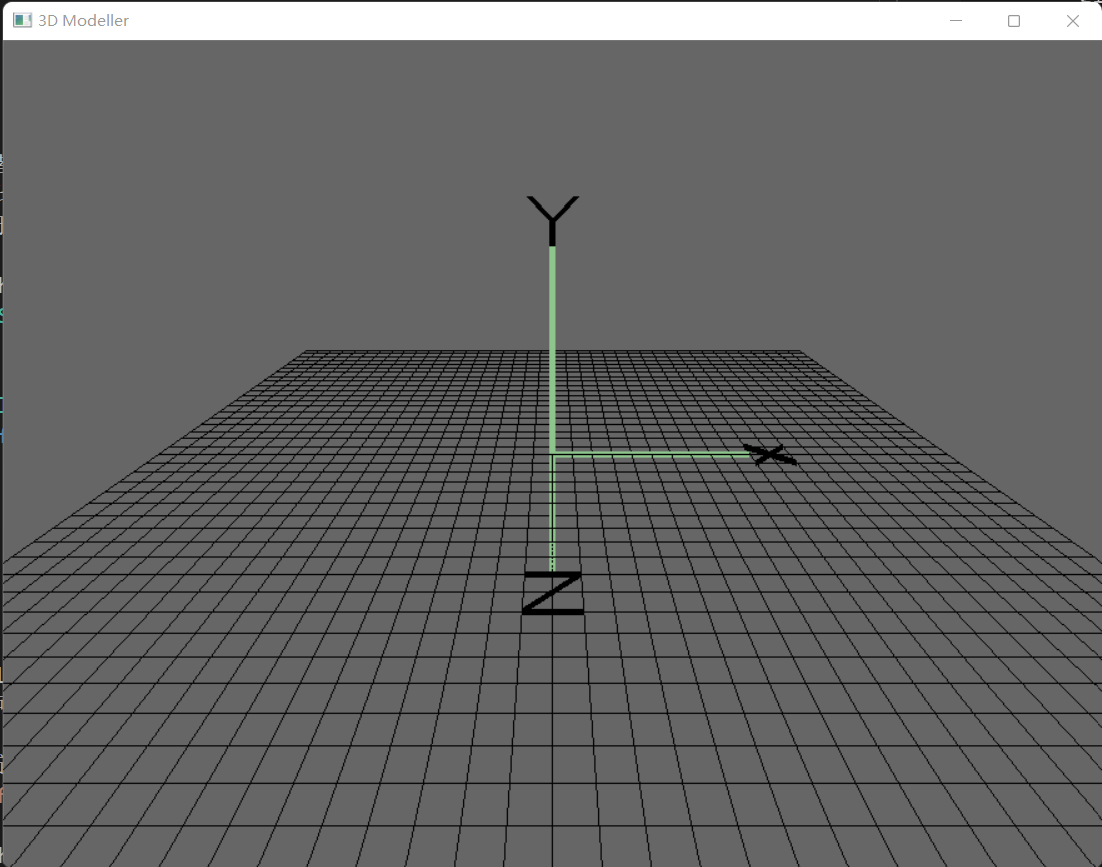### 步骤 2：定义交互形体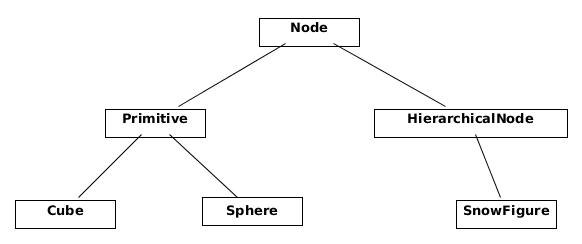plane
primitive
hierarchical

``````class Shape: pass
class Plane(Shape): pass
class ActiveMixin: pass
class Primitive(Shape, ActiveMixin): pass
class Cube(Primitivie): pass
class Sphere(Primitive): pass
``````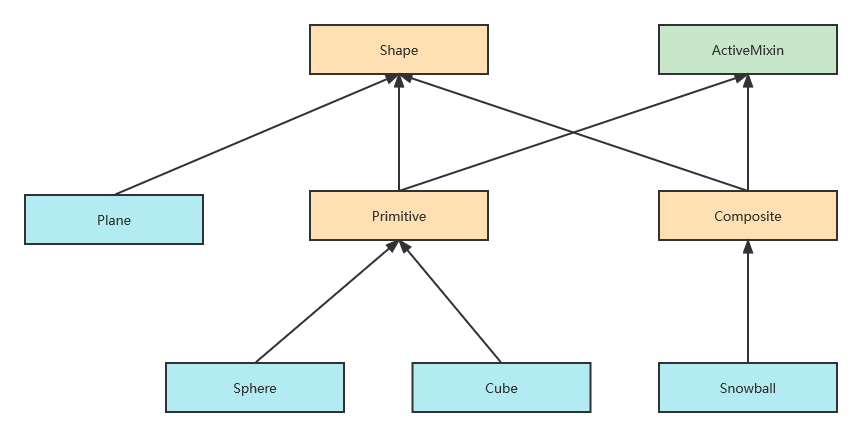`ActiveMixin` 用于支持可交互形体，也是我们这个步骤的重点。因为形体主要支持平移/缩放两种变换，所以我们添加成员变量以记录之：

``````class ActiveMixin:
def __init__(self):
self.translation = np.identity(4)
self.scaling = np.identity(4)

def translate(self, x, y, z):
self.translation = np.dot(self.translation, translation([x, y, z]))

def render(self):
glPushMatrix()
glMultMatrixf(np.transpose(self.translation))
glMultMatrixf(self.scaling)
self.render_self()
glPopMatrix()
``````

``````class Primitive(Shape, ActiveMixin):
def __init__(self, color_index=-1):
super().__init__()
if color_index >= 0:
self.color_index = color_index
else:
self.color_index = self.random_color_index()

def render_self(self):
color = self.COLORS[self.color_index]
glColor3f(color, color, color)
glCallList(self.id)
``````

``````class Sphere(Primitive):
id = 2

@classmethod
def define(cls):
glNewList(cls.id, GL_COMPILE)
glEndList()
``````

``````def define_shapes():
for cls_name, cls in globals().items():
if isinstance(cls, type) and issubclass(cls, Shape):
method = getattr(cls, 'define', None)
if method is not None:
method()
``````

``````class Scene:
def __init__(self):
self.shapes = [Plane()]

cube = Cube(color_index=1)
cube.translate(2, 0, 2)
self.shapes.append(cube)

sphere = Sphere(color_index=3)
sphere.translate(-2, 0, 2)
self.shapes.append(sphere)
``````

``````    def get_shapes(self, active):
return [x for x in self.shapes if x.is_active() == active]

def render(self):
...
glDisable(GL_LIGHTING)
for shape in self.get_shapes(False):
shape.render()

glEnable(GL_LIGHTING)
for shape in self.get_shapes(True):
shape.render()
...
``````

``````class Shape:
def is_active(self):
return isinstance(self, ActiveMixin)

class Scene:
def get_shapes(self, active):
return [x for x in self.shapes if x.is_active() == active]
``````

``````class GLWindow:
def __init__(self, pos, size, title):
...
self.scene = Scene()

def render(self):
self.scene.render()
``````

### 步骤 3：定义复合形体

``````class CompositeShape(Shape, ActiveMixin):
def __init__(self):
super().__init__()
self.children = []

def render_self(self):
for child in self.children:
child.render()
``````

``````class Snowball(CompositeShape):
def __init__(self):
super().__init__()
self.add_sphere((0, 0.1, 0), (0.8, 0.8, 0.8))
self.add_sphere((0, 0.75, 0), (0.7, 0.7, 0.7))
``````

`add_sphere(translation, scale)` 方法用于添加一个位置和缩放比例略有变化的球体。它的实现很直观，为节约篇幅，这里就不再列出了。为了看到效果，我们在 `add_sample_shapes()` 方法中添加一个雪球：

`````` def add_samle_shapes(self):
...
snowball = Snowball()
snowball.translate(-2, 0, -2)
self.shapes.append(snowball)
``````

### 步骤 4：基础性输入支持

``````class GLWindow:
def create_window(self, pos, size, title):
...
glutDisplayFunc(self.render)
glutIdleFunc(self.render)

glutMouseFunc(self.handle_mouse_button)
glutMotionFunc(self.handle_mouse_move)
glutKeyboardFunc(self.handle_keystroke)
glutSpecialFunc(self.handle_keystroke)

def normalize_pos(self, x, y):
width, height = glutGet(GLUT_WINDOW_WIDTH), glutGet(GLUT_WINDOW_HEIGHT)
return x, height - y

def handle_mouse_button(self, btn, mode, x, y):
print('mouse button:', btn, mode, x, y)

def handle_mouse_move(self, x, y):
print('mouse move:', x, y)

def handle_keystroke(self, key, x, y):
print('key stroke:', key, x, y)
``````

``````class GLWindow:
def __init__(self, pos, size, title):
...
self.mouse_btn = None
self.mouse_pos = None
``````

``````    def handle_mouse_button(self, btn, mode, x, y):
# print('mouse button:', btn, mode, x, y)
self.mouse_pos = self.normalize_pos(x, y)
if mode == GLUT_DOWN:
self.mouse_btn = btn
if btn == 3:  # scroll up
self.scene.translate(0, 0, 1.0)
elif btn == 4:  # scroll down
self.scene.translate(0, 0, -1.0)
else:  # mouse button up
self.mouse_btn = None
glutPostRedisplay()
``````

``````    def handle_mouse_move(self, x, y):
# print('mouse move:', x, y)
curr_pos = self.normalize_pos(x, y)
changed = False
if self.mouse_btn is not None:
dx = curr_pos - self.mouse_pos
dy = curr_pos - self.mouse_pos
if self.mouse_btn == GLUT_RIGHT_BUTTON:
self.scene.drag(curr_pos, (dx, dy))
elif self.mouse_btn == GLUT_MIDDLE_BUTTON:
self.scene.translate(dx / 60.0, dy / 60.0, 0)
changed = True
self.mouse_pos = curr_pos
if changed:
glutPostRedisplay()
``````

``````class Scene:
def drag(self, pos, delta):
self.trackball.drag_to(pos, pos, delta, delta)
``````

### 步骤 5：输入 AABB

`AABB` 是一种常见且简单的三维空间命中检测算法。它的基本思想是在目标形体设定一个最小的外接立方体，从鼠标点击位置发出一条假想的射线，只要射线击中了立方体，就认为点击命中。由于立方体的几何形状简单且规则，这种计算是非常高效的，对于比较规则的形体来说效果一般也很不错。当然，如果外接立方体与实际形体的差别很大，那么它的误差就会比较明显。所幸我们这里定义的都是非常规则的形体，因此采用 `AABB` 算法是合理的。对于复杂且要求较高的应用来说，可以探索其他更加复杂的命中算法。

``````class ActiveMixin:
def __init__(self):
...
self.aabb = self.define_aabb()

def pick(self, start, direction, mat):
newmat = np.dot(np.dot(mat, self.translation), np.linalg.inv(self.scaling))
results = self.aabb.ray_hit(start, direction, newmat)
return results
``````

``````    def pick(self, pos):
start, direction = self.get_ray(pos)
nearest_shape, nearest_distance = None, 2147483647  # sys.maxint
for shape in self.get_shapes(True):
hit, distance = shape.pick(start, direction, self.modelView)
if hit and distance < nearest_distance:
nearest_distance, nearest_shape = distance, shape

for child in self.get_shapes(True):
child.selected = (child is nearest_shape)
self.selected_shape = nearest_shape
``````

``````    def handle_mouse_button(self, btn, mode, x, y):
...
if mode == GLUT_DOWN:
...
if btn == GLUT_LEFT_BUTTON:
self.scene.pick(self.mouse_pos)
``````

``````    def handle_mouse_move(self, x, y):
...
if self.mouse_btn is not None:
if self.mouse_btn == GLUT_LEFT_BUTTON:
self.scene.move(curr_pos)
``````

`Scene.move()` 涉及到一些三维空间位置的计算，实现较为复杂，但逻辑是直观的。这里不再列出，有兴趣的同学可以阅读源码。

``````class ActiveMixin:
def render(self):
...
if self.selected:  # emit light if the node is selected
glMaterialfv(GL_FRONT, GL_EMISSION, [0.3, 0.3, 0.3])
self.render_self()
if self.selected:
glMaterialfv(GL_FRONT, GL_EMISSION, [0.0, 0.0, 0.0])
``````

### 步骤 6：输入其他

• `s/c/b` 键分别表示在当前位置增加一个球体/正方体/雪球；
• 键盘上下方向键对选中物体进行放大/缩小；
• 键盘左右键切换选中物体的颜色

``````    def handle_keystroke(self, key, x, y):
# print('key stroke:', key, x, y)
curr_pos = self.normalize_pos(x, y)
changed = True
if key == b's':
self.scene.place('sphere', curr_pos)
elif key == b'c':
self.scene.place('cube', curr_pos)
elif key == b'b':
self.scene.place('snowball', curr_pos)
elif key == 0x65:  # GLUT_KEY_UP
self.scene.scale_selected(True)
elif key == 0x67:  # GLUT_KEY_DOWN
self.scene.scale_selected(False)
elif key == 0x64:  # GLUT_KEY_LEFT
self.scene.rotate_color(True)
elif key == 0x66:  # GLUT_KEY_RIGHT
self.scene.rotate_color(False)
else:
changed = False
if changed:
glutPostRedisplay()
``````

``````    def place(self, type_name, pos):
start, direction = self.get_ray(pos)
new_shape = None
if type_name == 'sphere':
new_shape = Sphere()
elif type_name == 'cube':
new_shape = Cube()
elif type_name == 'snowball':
new_shape = Snowball()

if new_shape is not None:
translation = (start + direction * self.PLACE_DEPTH)
pre_tran = np.array([translation, translation, translation, 1])
translation = self.inverseModelView.dot(pre_tran)
new_shape.translate(translation, translation, translation)
self.shapes.append(new_shape)
``````

`Shape` 基类已经预定了一个可用的颜色列表。要切换颜色，我们添加一个辅助函数：

``````    def rotate_color(self, forward):
color_count = len(self.COLORS)
if forward:
self.color_index = (self.color_index + 1) % color_count
else:
self.color_index = (self.color_index + color_count - 1) % color_count
``````